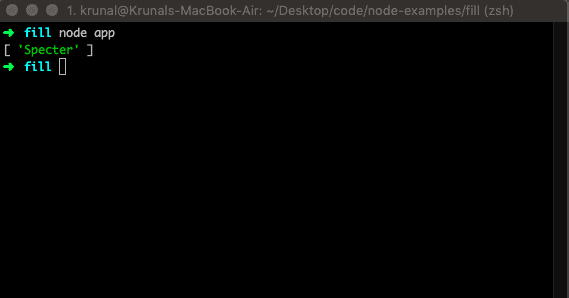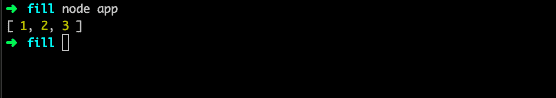# JavaScript Array Slice: How to Slice an Array

JavaScript slice() method returns the selected elements in an array as a new array object. The slice() method selects the elements starting at the given start argument and ends at, but omits the given end argument.

## JavaScript Array slice

JavaScript array slice() is a built-in function that returns the shallow copy of the portion of an array into a new array object selected from beginning to end.

The slice() method returns the selected elements in an array as a new array object. The original array will not be modified. So it is a pure function

The slice() method selects the elements starting at the given start argument and ends at, but omits the given end argument.

### Syntax

The syntax for the Javascript array slice() method is the following.

`array.slice(start, end)`

### Parameters

The start parameter is optional, and the integer specifies where to start the selection.

The end parameter is optional, and the integer specifies where to end the selection.

### Example

Let us take an example by creating a file called app.js and adding the following code.

```// app.js

let namepartner = ['Pearson', 'Specter', 'Litt'];
let suits = namepartner.slice(1, 2);

console.log(suits);```So here, what happens under the hood is that when the .slice() method is called frequently, this keyword works as an Array, then it iterates over an Array.

So one question in your mind is how this keyword in the .slice() functions as an Array. Because when you do.

`object.method()`

The object automatically refers to the value of this keyword in the javascript method(). So with:

`[1,2,4,5].slice()`

And the [1, 2, 4, 5] is an Array is set as the value of this in the .slice() method.

## Array-like objects

The slice() method can also be called to convert Array-like objects/collections into the new Array. You can bind a method to the object. The arguments inside the function are examples of the ‘array-like object.’

```// app.js

function list() {
return Array.prototype.slice.call(arguments);
}

const list1 = list(1, 2, 3);
console.log(list1);```

We can create a bindin with the .call() function of the Function.prototype method and we can also reduced using [].slice.call(arguments) instead of Array.prototype.slice.call().

The output of the following code is this.Let us take another example of the slice() method in Javascript.

```// app.js

let months = ['Jan', 'Feb', 'Mar', 'Apr', 'May', 'Jun', 'July', 'Aug', 'Sep'];
let cut = months.slice(2, 5);

console.log(cut);```

So, here the array index starts from 0. So we will get an array object with the values of [‘Mar’, ‘Apr’, ‘May’].

That’s it.

### 2 thoughts on “JavaScript Array Slice: How to Slice an Array”

1.But as someone who is only learning js, i’d like to point out that in this case is good to explain why the result is what it is.
let namepartner = [‘Pearson’, ‘Specter’, ‘Litt’];
let suits = namepartner.slice(1, 2);

console.log(suits);
Specter

slice method with parameters 1 and 2 starts at 1 and end at 2(2 not included).
so as arrays start count as 0, Pearson is ignored, Spencer with position 1 is the result and Litt with position 2 is also ignored.

also maybe better example would be bigger array.

2.